Need Help?

Subscribe to Statics

###### \${selected_topic_name}
• Notes

Draw the free-body diagram of member $A B,$  which is  supported by a roller at  $A$ and a pin at  $B$ . Explain the  significance of each force on the diagram. (See Fig. } 5-7 b .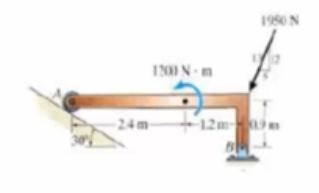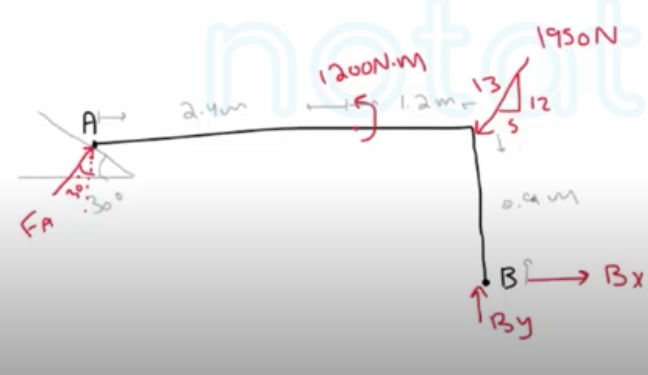Determine the horizontal and vertical components  of reaction at the pin  $A$  and the tension developed in cable $B C$ used to support the stecl frame.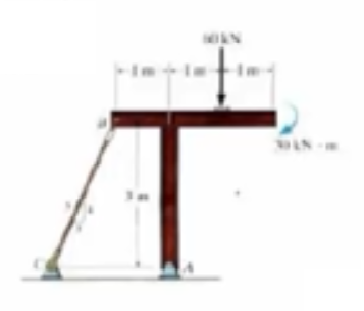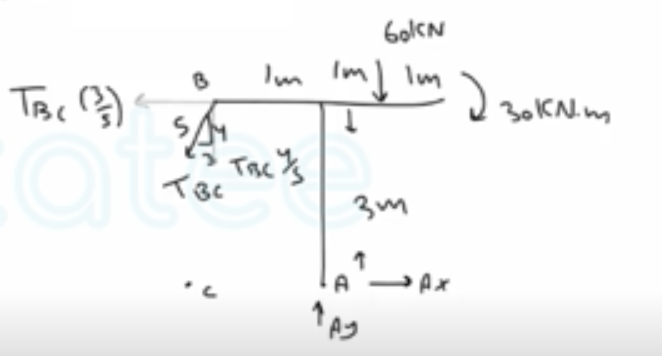$\sum M_{A}=0$

$T_{BC}\left(\frac{4}{5}\right)(1)+T_{BC}\left(\frac{3}{5}\right)(3)-60(1)-30=0$

$∴ T_{B C}=34.62 \mathrm{kN}$

$\stackrel{+}\longrightarrow \sum F_{x}=0$

$A_X-T_{BC}(\frac{3}{5})=0$

$∴ A _X=20.77 \mathrm{kN} \rightarrow$

$+\uparrow \sum F_ y=0$

$A_y-60-T_{BC}\left(\frac{4}{5}\right)=0$

$∴ A_ y=87.7 \mathrm{kN}\quad\uparrow$

The horizontal beam is supported by springs at its ends. Each spring has a stiffness of $k=5 \mathrm{kN} / \mathrm{m}$  and is originally unstretched so that the beam is in the horizontal  position. Determine the angle of tilt of the beam if a load of $800 \mathrm{N}$  is applied at point  $C$  as shown.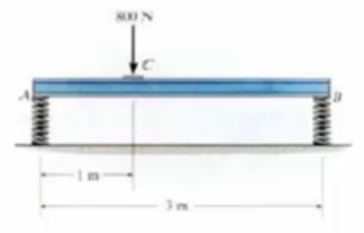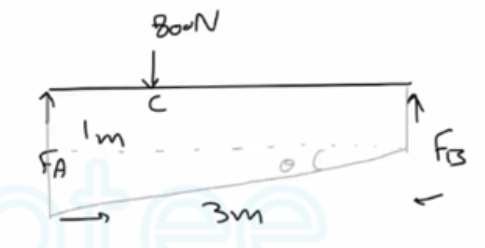$+\uparrow \sum F _y=0$

$F_{A}+C_{B}=800 N$

$\sum M_{A}=0.$

$F_{B}(3)-800(1)=0$

$∴ F_{B}=267 \mathrm{N}$

$F_{A}=800-F_{B}=800-267=533 N$

$F=k _s \quad \longrightarrow \begin{array}{l}{S_{A}=\frac{F_{A}}{k}=\frac{533}{5}=0.107 \mathrm{m}} \\ {S_{B}=\frac{F_{B}}{K}=\frac{267}{5}=0.053 \mathrm{m}}\end{array}$

$\theta=\tan ^{-1}\left(\frac{(S_ A-5 _B)}{3}\right)$

$=1.02^{\circ}$